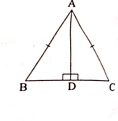"
">

# $\triangle A B C$ is an isosceles triangle such that $A B=A C$, $A D \perp B C$a) Prove that $\triangle A B D \cong \triangle A C D$b) Prove that $\angle B=\angle C$c) Is D the mid-point of BC?"

Given :

$AB = AC$

$AD$ is perpendicular to $BC$.

To do :

a) Prove that $\triangle A B D \cong \triangle A C D$

b) Prove that $\angle B=\angle C$

c) Is D the mid-point of BC?

Solution :

RHS Congruence:

In two right-angled triangles, if the length of the hypotenuse and one side of one triangle, is equal to the length of the hypotenuse and corresponding side of the other triangle, then the two triangles are congruent.

$AD$ is perpendicular to $BC$.

$∠ADB = ∠ADC = 90°$

(a)

In $△ABD$ and $△ACD$,

$AB = AC$  (Given)

$AD = AD$ (Common side)

$∠ADB = ∠ADC = 90°$

Therefore, by RHS congruence,

$△ABD ≅ △ACD$

Hence proved.

(b)

$△ABD ≅ △ACD$,

This implies,

$∠ABD = ∠ACD$ (CPCT)

Hence proved.

(c)

$△ABD ≅ △ACD$,

This implies,

$BD = DC$  (CPCT)

Therefore,

D is the mid-point of BC.

Updated on: 10-Oct-2022

29 Views